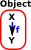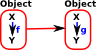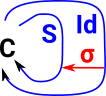# Maths - Functor CategoryIn a functor category the object is a functor.Morphisms are structure preserving maps between these functors.
 Since the objects are all functors from X to Y we can redraw it like this:So we can the that these morphisms are really natural transformations (as described on page here).

So a functor category is a category where:

• The objects are functors.
• The morphisms are natural transformations.

#### Example of a Functor Category

See presheaves on page here.

## Endofunctor Category

In addition to the stucture in the endofunctor we also get structure because endofunctors compose.

This gives a monoidal category where the binary operation is the composition of the endofunctors and the unit is the Id functor.

• The binary operation is a tensor product.
• The unit is the Id functor.

What are the initial and terminal objects in this category?

Is the teminal object the Id functor? (this thread says no).

## Pointed Endofunctor

 It is well pointed if: S σ = σ S## Monoidal Category

The above endofunctor category is a monoidal category.

## Related Categories

Where I can, I have put links to Amazon for books that are relevant to the subject, click on the appropriate country flag to get more details of the book or to buy it from them.The Princeton Companion to Mathematics - This is a big book that attempts to give a wide overview of the whole of mathematics, inevitably there are many things missing, but it gives a good insight into the history, concepts, branches, theorems and wider perspective of mathematics. It is well written and, if you are interested in maths, this is the type of book where you can open a page at random and find something interesting to read. To some extent it can be used as a reference book, although it doesn't have tables of formula for trig functions and so on, but where it is most useful is when you want to read about various topics to find out which topics are interesting and relevant to you.